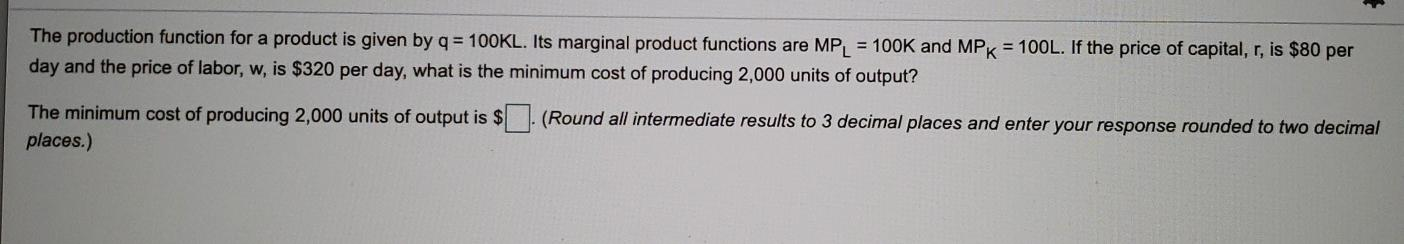### Create an Account

Already have account?

### Forgot Your Password ?

Home / Questions / The production function for a product is given by q = 100KL. Its marginal product function...

# The production function for a product is given by q = 100KL. Its marginal product functions are MPL = 100K and MPK = 100L. If the price of capital, r, is \$80 per day and the price of labor,

The production function for a product is given by q = 100KL. Its marginal product functions are MPL = 100K and MPK = 100L. If the price of capital, r, is \$80 per day and the price of labor, w, is \$320 per day, what is the minimum cost of producing 2,000 units of output? The minimum cost of producing 2,000 units of output is \$ places.) (Round all intermediate results to 3 decimal places and enter your response rounded to two decimalJun 10 2021 View more View Less

#### Answer (Solved)Subscribe To Get Solution